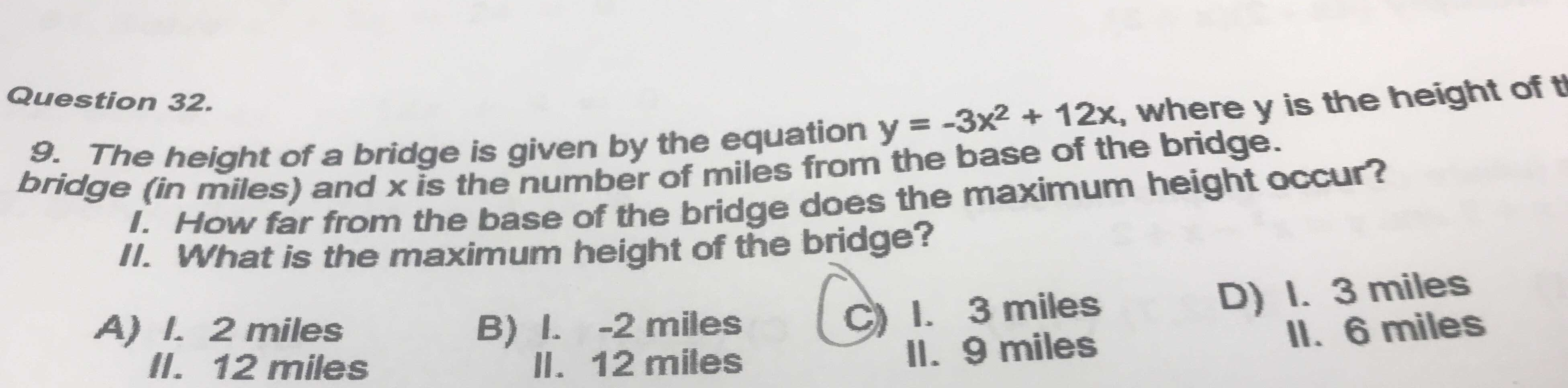### Still have math questions?

Algebra
QuestionThe height of a bridge is given by the equation $$y = - 3 x ^ { 2 } + 12 x$$ , where $$y$$ is the height of the bridge (in miles) and $$x$$ is the number of miles from the base of the bridge. I. How far from the base of the bridge does the maximum height occur? II. What is the maximum height of the bridge?

A) I. 2miles

II. $$12$$ miles

B) I. -2miles

II. $$12$$ miles

C) I. 3miles

II. 9miles

D) I. 3miles

II. 9 miles